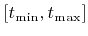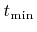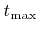Next: C. Inequalities Up: B. Ray-Isosurface Intersection Previous: B.1 Setup of the

# B.2 Root Finding

Once the polynomial is set up for a grid cell, it can be tested for any ray-surface intersection within its interior. Letbe the set of parameter values, for which the ray lies inside the corresponding grid cell. If there exists any real root in this interval, the ray intersects the surface. In this case the smallest real root, which corresponds to the first surface intersection, must be evaluated.

To check, if there is a real root in, the extrema of the polynomial are first analyzed, as proposed in . The extrema can easily be determined and evaluated using analytical expressions for all quadratic and cubic polynomials. The signs of the extremal values and the function values atanddetermine whether there exists a real root in, and, if this is the case, they allow for refinement of the potential solution set. The smallest root can then be found using standard root finding techniques. A hybrid method, which combines bisection and the Newton-Raphson method , was used in this work.Next: C. Inequalities Up: B. Ray-Isosurface Intersection Previous: B.1 Setup of the

Otmar Ertl: Numerical Methods for Topography Simulation